# ordfilt2

2-D order-statistic filtering

## Syntax

``B = ordfilt2(A,order,domain)``
``B = ordfilt2(A,order,domain,S)``
``B = ordfilt2(___,padopt)``

## Description

example

````B = ordfilt2(A,order,domain)` replaces each element in `A` by the `order`th element in the sorted set of neighbors specified by the nonzero elements in `domain`. ```
````B = ordfilt2(A,order,domain,S)` filters `A`, where `ordfilt2` uses the values of `S` corresponding to the nonzero values of `domain` as additive offsets. You can use this syntax to implement grayscale morphological operations, including grayscale dilation and erosion.```
````B = ordfilt2(___,padopt)` filters `A`, where `padopt` specifies how `ordfilt2` pads the matrix boundaries.```

## Examples

collapse all

Read image into workspace and display it.

```A = imread('snowflakes.png'); figure imshow(A)```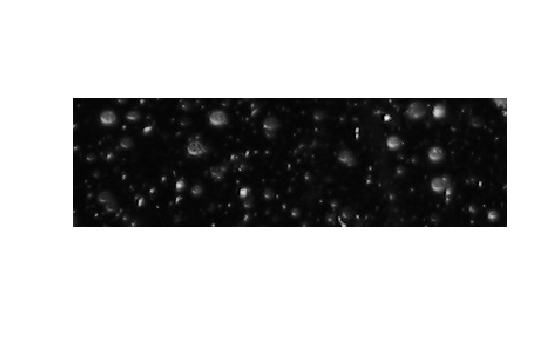Filter the image and display the result.

```B = ordfilt2(A,25,true(5)); figure imshow(B)```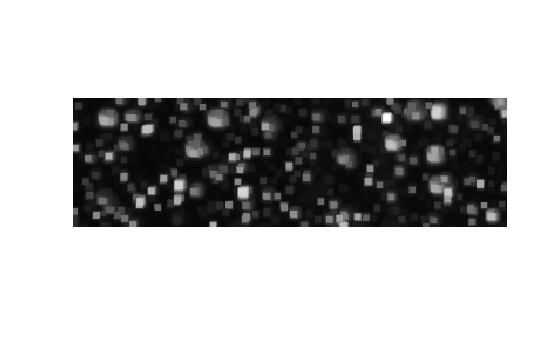## Input Arguments

collapse all

Data to filter, specified as a 2-D numeric matrix or 2-D logical matrix.

Data Types: `single` | `double` | `int8` | `int16` | `int32` | `uint8` | `uint16` | `uint32` | `logical`

Element to replace the target pixel, specified as a real scalar integer.

Data Types: `double`

Neighborhood, specified as a numeric or logical matrix containing `1`s and `0`s. `domain` is equivalent to the structuring element used for binary image operations. The `1`-valued elements define the neighborhood for the filtering operation. The table gives examples of some common filters.

Type of Filtering OperationMATLAB codeNeighborhoodSample Image Data, Indicating Selected Element
Median filter`B = ordfilt2(A,5,ones(3,3))`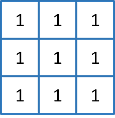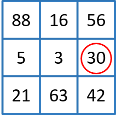Minimum filter`B = ordfilt2(A,1,ones(3,3))`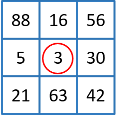Maximum filter`B = ordfilt2(A,9,ones(3,3))`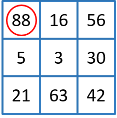Minimum of north, east, south, and west neighbors```B = ordfilt2(A,1,[0 1 0; 1 0 1; 0 1 0])```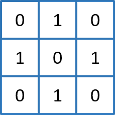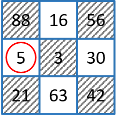Data Types: `single` | `double` | `int8` | `int16` | `int32` | `int64` | `uint8` | `uint16` | `uint32` | `uint64` | `logical`

Additive offsets, specified as a numeric matrix of the same size as `domain`.

Data Types: `single` | `double` | `int8` | `int16` | `int32` | `int64` | `uint8` | `uint16` | `uint32` | `uint64` | `logical`

Padding option, specified as one of the following values.

OptionDescription
`'zeros'`Pad array boundaries with `0`’s.
`'symmetric'`

Pad array with mirror reflections of itself.

Data Types: `char` | `string`

## Output Arguments

collapse all

Filtered data, returned as a 2-D numeric matrix or 2-D logical matrix of the same class as the input data `A`.

## Tips

• When working with large domain matrices that do not contain any zero-valued elements, `ordfilt2` can achieve higher performance if `A` is in an integer data format (`uint8`, `int8`, `uint16`, `int16`). The gain in speed is larger for `uint8` and `int8` than for the 16-bit data types. For 8-bit data formats, the domain matrix must contain seven or more rows. For 16-bit data formats, the domain matrix must contain three or more rows and 520 or more elements.

 Haralick, Robert M., and Linda G. Shapiro, Computer and Robot Vision, Volume I, Addison-Wesley, 1992.

 Huang, T.S., G.J.Yang, and G.Y.Tang. "A fast two-dimensional median filtering algorithm.", IEEE transactions on Acoustics, Speech and Signal Processing, Vol ASSP 27, No. 1, February 1979